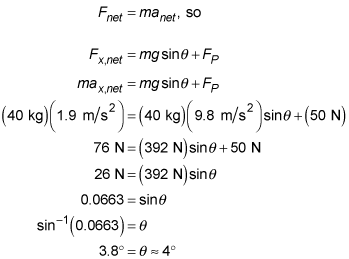##### Physics I: 501 Practice Problems For Dummies (+ Free Online Practice)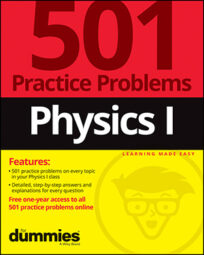In physics, if an object is pushed down a slope, the slope's angle of inclination affects the object's acceleration. For example, when you push a sled down an ice-covered hill, you can calculate the hill's angle of inclination given the sled's mass and acceleration.

Weight is the gravitational force that Earth exerts on an object. Near the surface of Earth, weight is equal to mass times 9.8 meters per second squared. The magnitude of the force of friction is equal to the coefficient of friction times the magnitude of the normal force. Friction always opposes the relative motion between two surfaces.

Here are some practice questions to help illustrate this concept.

## Practice questions

1. A 25-kilogram sled slides down a hill of ice-covered snow. If the sled accelerates down the hill at a rate of 4.8 meters per second squared, what is the hill's angle of inclination with respect to the horizontal?

Assume the snow is a frictionless surface and round your answer to the nearest whole degree.

2. Samantha pushes her daughter, who is sitting on a sled, down a snowy hill of constant slope with a force of 50 newtons. The sled accelerates at 1.9 meters per second squared. If the combined mass of her daughter and the sled is 40 kilograms, what is the hill's angle of inclination?

The following are the answers to the practice questions:

1. 29 degrees.

First draw the free-body diagram: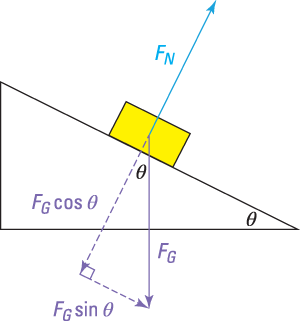Only one force is pointing along the axis of motion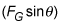so that's the only contributor to the net acceleration along the hill's surface: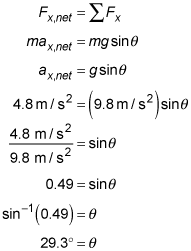2. 4 degrees.

Three forces are involved (FN, the normal force; FG, the force due to Earth's gravity; and FP, the force from Samantha's push). Draw a free-body diagram showing the vectors and any necessary components to align the forces into two perpendicular axes: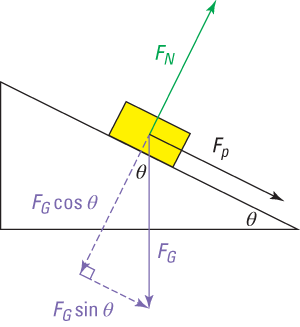The sled moves along the axis parallel to the hill's surface, so that's the direction of the acceleration you need to examine. Adding up the forces in that direction: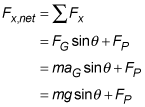(This setup uses "down the slope" as the positive direction.) By Newton's second law,